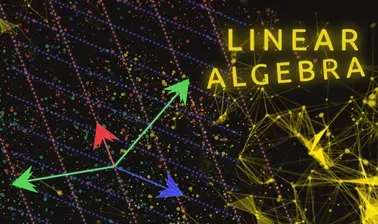There is 0 item now in your comparison listView a comparison list

# Linear Algebra, Part 1

Price
Free
12,452 people completed this program

## Overview

Linear algebra is at the core of all of modern mathematics, and is used everywhere from statistics and data science, to economics, physics and electrical engineering. However, learning the subject is not principally about acquiring computational ability, but is more a matter of fluency in its language and theory.

In this course, we will start with systems of linear equations, and connect them to vectors and vector spaces, matrices, and linear transformations. We will be emphasizing the vocabulary throughout, so that students become comfortable working with the different aspects.

We will then introduce matrix and vector operations such as matrix multiplication and inverses, paying particular attention to their underlying purposes. Students will learn not just how to calculate them, but also why they work the way that they do.

We willdiscuss the key concepts of basis and dimension, which form the foundation for many of the more advanced concepts of linear algebra.

The last chapter concerns inner products, which allow us to use linear algebra for approximating solutions; we will see how this allows for applications ranging from statistics and linear regression to digital audio.

The relationships between linear equations, matrices, and linear transformations; the principles of vector and matrix operations; the significance of basis and dimension of a vector space; the applications of inner products and orthogonality.

Rice University

## Reviews(0)

Help others make their choice. Be the first one to leave a review

Leave a review